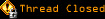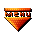# Topic awaiting preservation: Calculation problem (ASP) (Page 1 of 1)Hiroki
Paranoid (IV) Inmate

From: NZ
Insane since: Dec 2002posted 11-05-2003 00:38

I am trying to calculate using ASP.

Here is my code:

code:
```If Request.Form("cal") = "Calculation" Then
Dim q,p
q = Request.Form("quant")
p = Request.Form("price")
Response.write(q)
Response.Write(p)
Response.write(q*p)
End IF```

When user click calculation button, cost have to be printed on the screen.
But this line:

Response.Write(q*p)

it is causing error.
I don't know why cuz I did tutorial and got a code like this:

code:
```<%
sub vbproc(num1,num2)
response.write(num1*num2)
end sub
%>```

Hiroki Kozai

Rhino
Bipolar (III) Inmate

From: New Jersey, USA
Insane since: Jul 2003posted 11-10-2003 10:26

Try converting the returned values into Numbers !